﻿ GPT3模型在安徽地区的性能文章快速检索 高级检索
 大地测量与地球动力学2023, Vol. 43Issue (5): 481-486  DOI: 10.14075/j.jgg.2023.05.008### 引用本文QI Zimin, QU Xiaochuan, LAI Shandong, et al. Performance of GPT3 Model in Anhui Province[J]. Journal of Geodesy and Geodynamics, 2023, 43(5): 481-486.### Foundation support

National Natural Science Foundation of China, No.42171141.

### Corresponding author

QU Xiaochuan, lecture, majors in GNSS atmosphere, E-mail: qqxxcc@hfut.edu.cn.

### 第一作者简介

QI Zimin, postgraduate, majors in GNSS atmosphere, E-mail: 1085308120@qq.com.

### 文章历史

GPT3模型在安徽地区的性能

1. 合肥工业大学土木与水利工程学院，合肥市屯溪路193号，230009;
2. 江西省自然资源测绘与监测院，南昌市站前西路159号，330009

1 GPT3模型

GPT3模型可以提供1°×1°与5°×5°两种格网分辨率的气压、温度、加权平均温度、水汽递减因子等气象参数。顾及各气象参数的周年、半周年变化项，可表示为

 $\begin{gathered}y(t)=A_0+A_1 \cos \left(2 \pi \frac{\text { doy }}{365.25}\right)+ \\ A_2 \sin \left(2 \pi \frac{\text { doy }}{365.25}\right)+ \\ A_3 \cos \left(4 \pi \frac{\text { doy }}{365.25}\right)+A_4 \sin \left(4 \pi \frac{\text { doy }}{365.25}\right)\end{gathered}$ (1)

 \left\{\begin{aligned} T & =T_0+\mathrm{d} T \mathrm{~d} h \\ P & =P_0 \exp \\ & \left\{-g_m \frac{\mathrm{d} M_{\mathrm{tr}}}{R_g T_0(1+0.6077 Q)} \mathrm{d} h\right\} \\ e_0 & =Q \frac{P}{0.622+0.378 Q} \\ e & =e_0\left(100 \frac{P}{P_0}\right)^{\lambda+1}\end{aligned}\right. (2)

2 数据及方法 2.1 数据来源表 1 数据处理策略 Tab. 1 Data processing strategy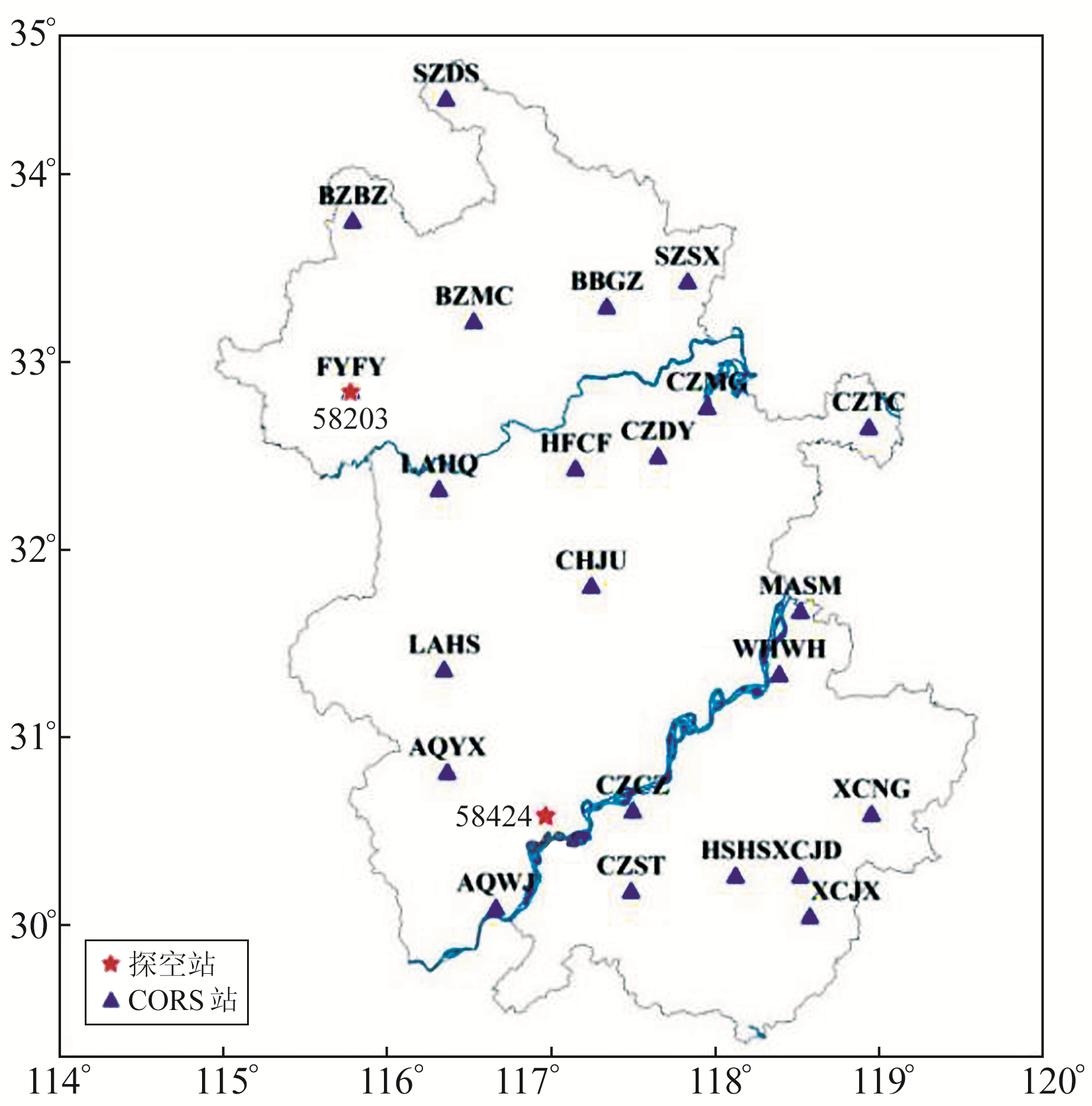图 1 CORS站与探空站分布 Fig. 1 Distribution of CORS stations and radiosonde stations
2.2 计算方法

2.2.1 Tm计算

Tm是反演PWV必不可少的关键参数之一，可由数值积分法计算得到：

 $T_m=\frac{\int \frac{e}{T} \mathrm{~d} h}{\int \frac{e}{T^2} \mathrm{~d} h}=\frac{\sum\limits_{i=1}^n\left(\frac{e_i}{T_i}\right) \Delta h_i}{\sum\limits_{i=1}^n\left(\frac{e_i}{T_i^2}\right) \Delta h_i}$ (3)

 $e=6.112 \times \exp (17.62 t /(243.12+t))$ (4)

2.2.2 GNSS-PWV计算

 $\left\{\begin{array}{l}\mathrm{PWV}=\varPi \times \mathrm{ZWD} \\ \varPi=\frac{10^6}{\rho_w R_v\left(k_3 / T_m+k_2^{\prime}\right)}\end{array}\right.$ (5)

2.2.3 探空站PWV计算

 $\left\{\begin{array}{l}\mathrm{PWV}=\frac{1}{g_m} \int_{P_z}^{P_0} q \mathrm{~d} p \\ q=\frac{622 e}{p-0.378 e}\end{array}\right.$ (6)

3 结果与分析 3.1 GPT3模型的ZTD精度评估图 2 PPP-ZTD和GPT3-ZTD时间序列 Fig. 2 PPP-ZTD and GPT3-ZTD time series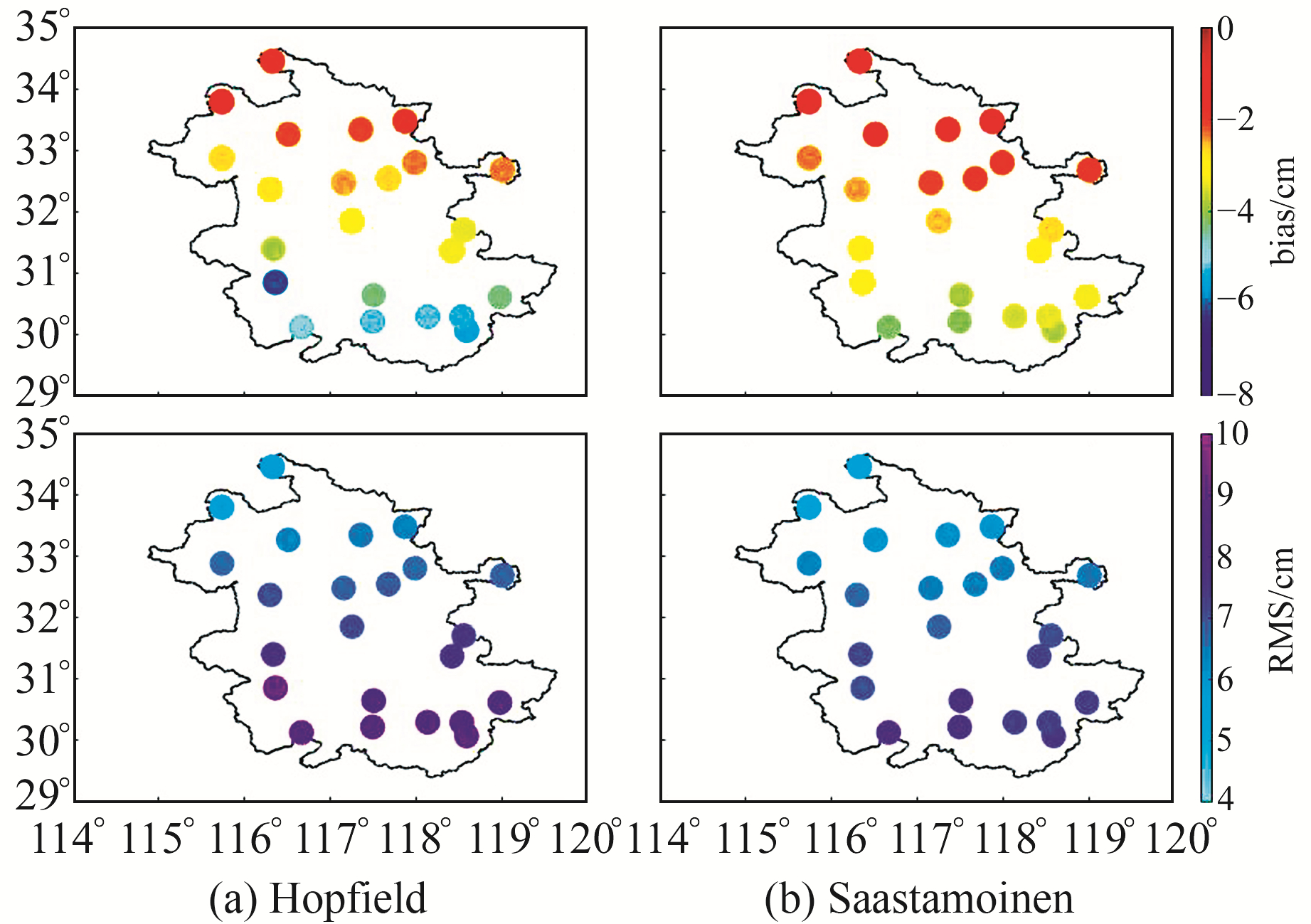图 3 安徽省23个CORS站的GPT3模型精度 Fig. 3 Accuracy of GPT3 model at 23 CORS stations in Anhui province表 2 不同对流层模型的bias和RMS Tab. 2 bias and RMS of different tropospheric models表 3 组合模型在不同季节的精度信息 Tab. 3 Precision of combination models in different seasons
3.2 GPT3模型的PWV精度评估 3.2.1 Tm精度评估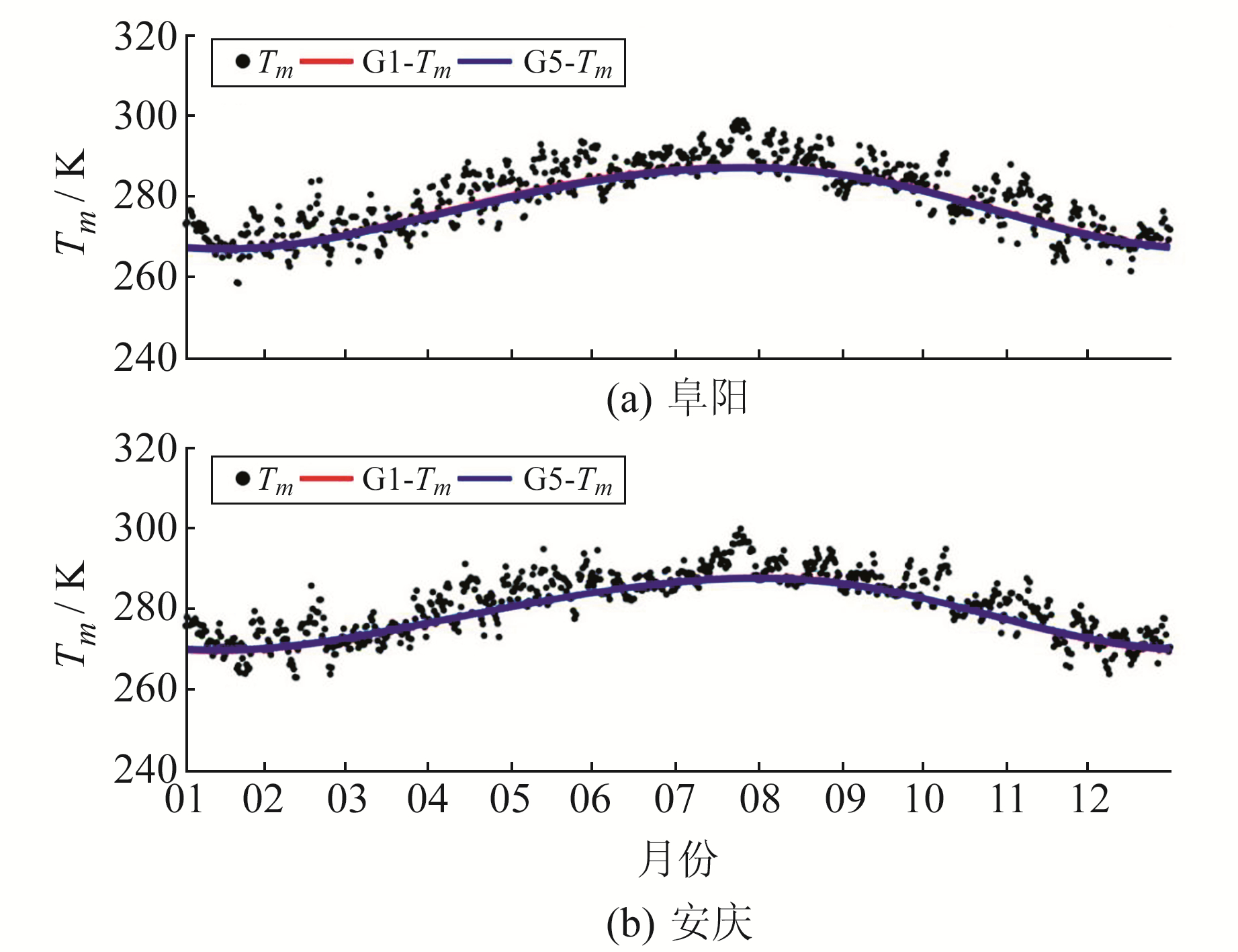图 4 2种格网分辨率的GPT3模型Tm时序分布 Fig. 4 Tm time series of GPT3 model with two grid resolutions表 4 2种分辨率Tm的精度统计 Tab. 4 Accuracy statistics of two resolutions of Tm
3.2.2 PWV精度评估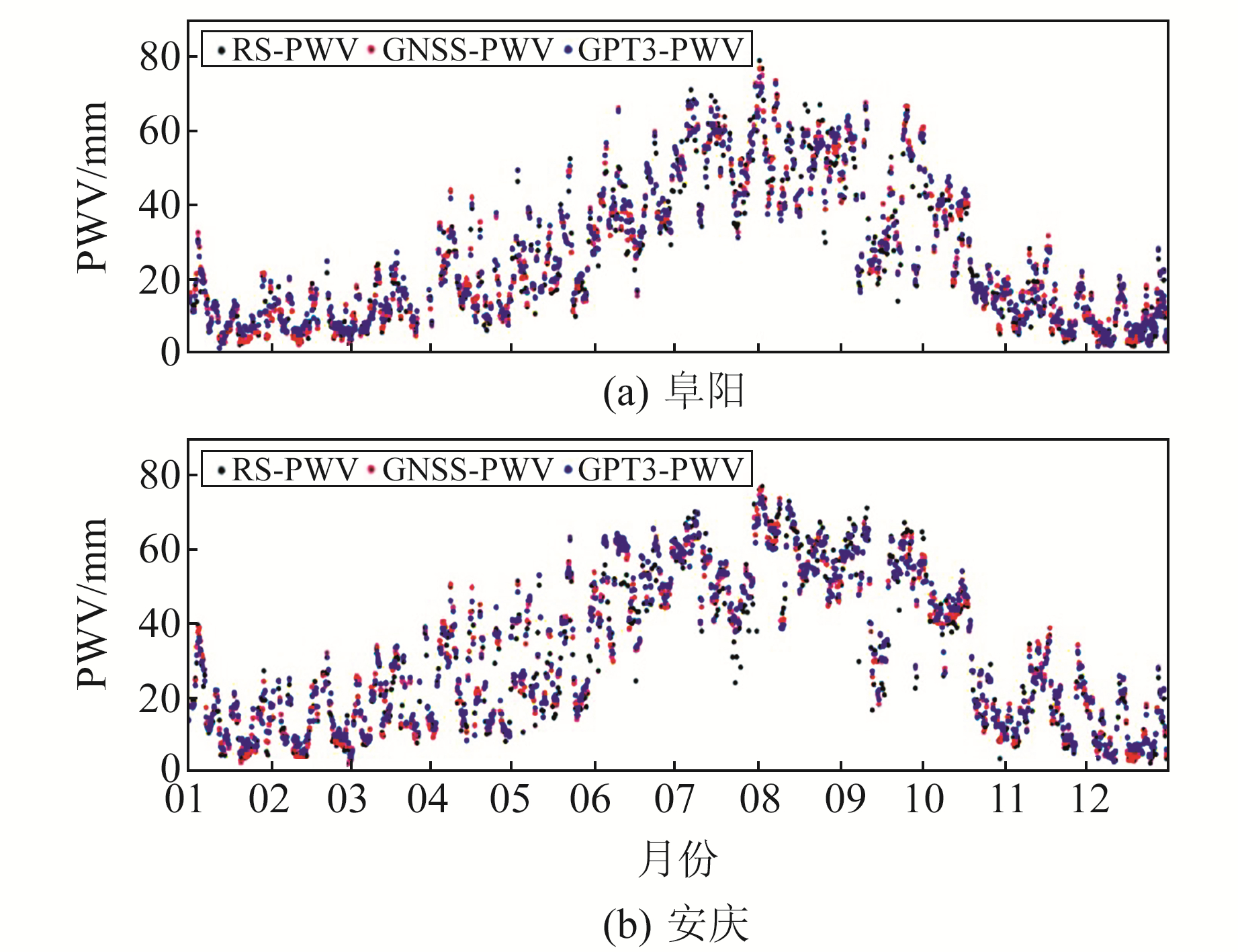图 5 安庆站和阜阳站的PWV Fig. 5 PWV at FYFY and AQWJ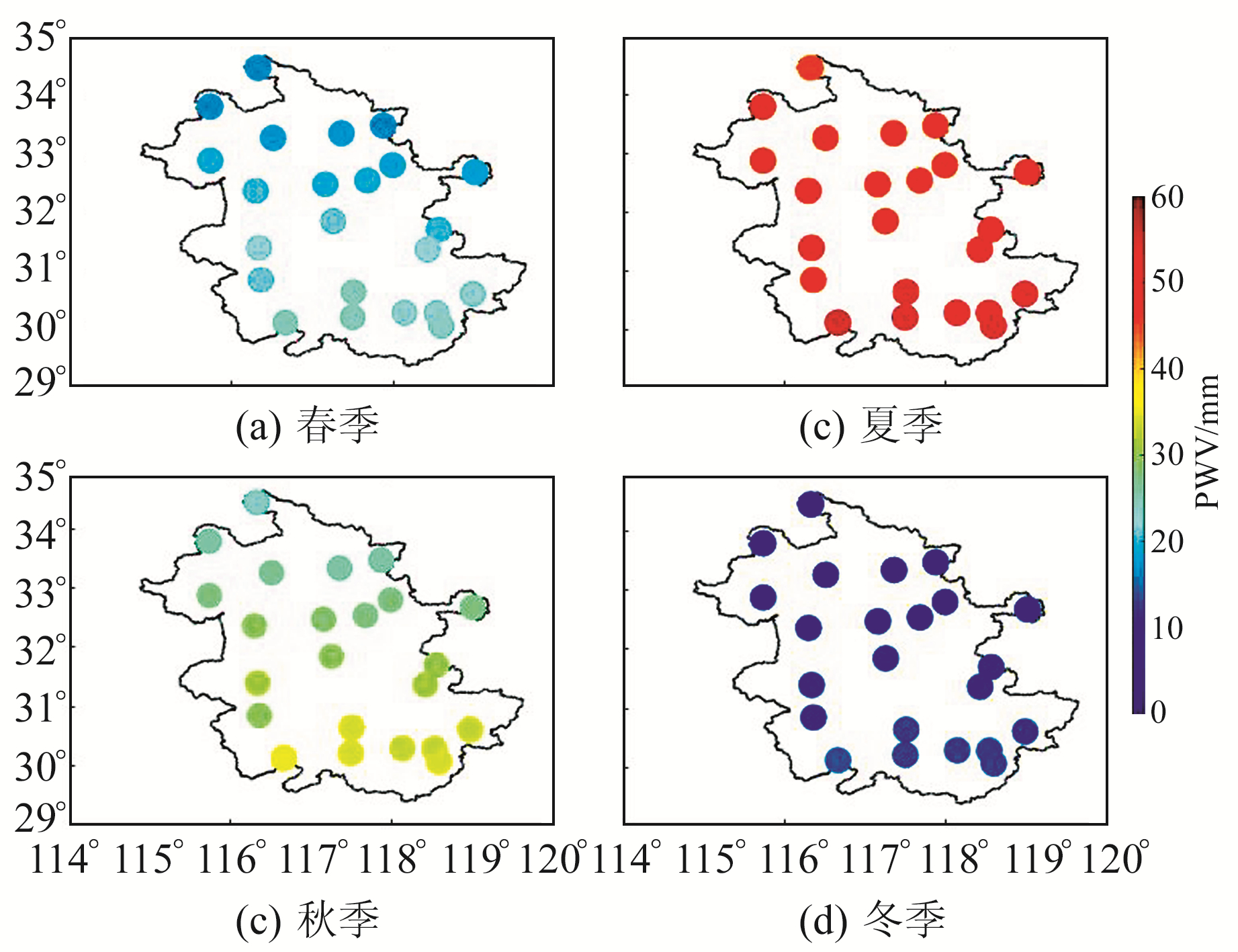图 6 GPT3-PWV的时空分布 Fig. 6 Spatio-temporal distribution of GPT3-PWV表 5 PWV精度统计 Tab. 5 PWV accuracy statistics
4 结语

1) GPT3模型的反演精度在安徽区域具有明显的时空分布特征，ZTD精度自北向南逐渐降低，且春、冬季精度优于夏、秋季。同时，GPT3模型普遍低估了安徽地区的ZTD。

2) 在相同气象参数条件下，GPT3+Sasstamoinen组合模型的ZTD精度要优于GPT3+Hopfield，二者的差异在皖北较小，但自北向南逐渐变大，进一步反映Saastamoinen模型比Hopfield模型更适用于安徽地区。

3) 在安徽地区，GPT3模型2种格网分辨率的Tm精度基本相当，平均偏差在-2.0 K左右，RMS在4.5 K左右。

4) 基于GPT3模型气象参数反演的PWV与探空站的PWV具有较高的一致性，GPT3-PWV在空间上由皖南向皖北逐渐减小，且夏季最大、冬季最小。PWV精度表现出明显的季节性特征，春、冬季的精度优于夏、秋季。GPT3-PWV的精度较高，仅比GNSS-PWV略低0.3~0.5 mm。

  Saastamoinen J. Introduction to Practical Computation of Astronomical Refraction[J]. Bulletin Géodésique, 1972, 106(1): 383-397 DOI:10.1007/BF02522047 (0)  Hopfield H S. Tropospheric Effect on Electromagnetically Measured Range: Prediction from Surface Weather Data[J]. Radio Science, 1971, 6(3): 357-367 DOI:10.1029/RS006i003p00357 (0)  Böhm J, Heinkelmann R, Schuh H. Short Note: A Global Model of Pressure and Temperature for Geodetic Applications[J]. Journal of Geodesy, 2007, 81(10): 679-683 DOI:10.1007/s00190-007-0135-3 (0)  Lagler K, Schindelegger M, Böhm J, et al. GPT2: Empirical Slant Delay Model for Radio Space Geodetic Techniques[J]. Geophysical Research Letters, 2013, 40(6): 1 069-1 073 DOI:10.1002/grl.50288 (0)  Böhm J, Möller G, Schindelegger M, et al. Development of an Improved Empirical Model for Slant Delays in the Troposphere(GPT2w)[J]. GPS Solutions, 2015, 19: 433-441 DOI:10.1007/s10291-014-0403-7 (0)  Landskron D, Böhm J. VMF3/GPT3: Refined Discrete and Empirical Troposphere Mapping Functions[J]. Journal of Geodesy, 2018, 92(4): 349-360 DOI:10.1007/s00190-017-1066-2 (0)  黄良珂, 李琛, 黄军胜, 等. 广西地区GPT2w对流层延迟模型适用性分析[J]. 桂林理工大学学报, 2018, 38(2): 289-294 (Huang Liangke, Li Chen, Huang Junsheng, et al. Assessment of GPT2w Tropospheric Delay Model over Guangxi[J]. Journal of Guilin University of Technology, 2018, 38(2): 289-294) (0)  蔡猛, 刘立龙, 黄良珂, 等. GPT3模型反演GNSS大气可降水量精度评定[J]. 大地测量与地球动力学, 2022, 42(5): 483-488 (Cai Meng, Liu Lilong, Huang Liangke, et al. Evaluation of GNSS Precipitable Water Vapor Derived from GPT3 Model[J]. Journal of Geodesy and Geodynamics, 2022, 42(5): 483-488) (0)  黄聪, 郭杭. 基于GPT3模型的ZTD及PWV反演精度分析[J]. 大地测量与地球动力学, 2022, 42(5): 489-493 (Huang Cong, Guo Hang. Accuracy Analysis of ZTD and Precipitable Water Vapor Inversion Based on GPT3 Model[J]. Journal of Geodesy and Geodynamics, 2022, 42(5): 489-493) (0)  Geng J H, Yang S F, Guo J. Assessing IGS GPS/Galileo/BDS-2/BDS-3 Phase Bias Products with PRIDE PPP-AR[J]. Satellite Navigation, 2021, 2(1): 238-252 (0)  Geng J H, Wen Q, Zhang Q Y, et al. GNSS Observable-Specific Phase Biases for All-Frequency PPP Ambiguity Resolution[J]. Journal of Geodesy, 2022, 96(2) (0)  王秋龙. 安徽省近50余年降水量时空变化特征分析[J]. 测绘工程, 2014, 23(11): 19-24 (Wang Qiulong. Temporal and Spatial Distribution of Precipitation over Anhui Province in Recent 50 Years[J]. Engineering of Surveying and Mapping, 2014, 23(11): 19-24) (0)
Performance of GPT3 Model in Anhui Province
QI Zimin1QU Xiaochuan1LAI Shandong2     GAO Fei1     YU Liangbin1
1. College of Civil Engineering, Hefei University of Technology, 193 Tunxi Road, Hefei 230009, China;
2. Jiangxi Provincial Institute of Natural Resource Surveying and Monitoring, 159 West-Zhanqian Road, Nanchang 330009, China
Abstract: Based on the zenith tropospheric delay(ZTD) calculated from the data of 23 continuously operating reference stations(CORS) in Anhui province, we analyze the applicability of two tropospheric model schemes, GPT3+Hopfield and GPT3+Sasstamoinen tamoinen. Using sounding data, we analyzed the accuracy of GPT3 model in estimating weighted average temperature (Tm) and the inversion accuracy of the precipitable water vapor (PWV). The results show that: 1) the ZTD accuracy of GPT3 combined with Saastamoinen model is better than that of Hopfield model. At the same time, the ZTD accuracy of GPT3 model has significant spatial-temporal distribution characteristics. The accuracy of southern Anhui is lower than that of northern Anhui, and the accuracy in spring and winter is better than in summer and autumn; 2) In Anhui province, the Tm accuracy of the two grid resolutions of GPT3 model are basically the same; the average deviation is about -2.0 K, and the RMS value is about 4.5 K; 3) In Anhui province, the PWV (GPT3-PWV) inversed based on the meteorological parameters of the GPT3 model has high consistency with the PWV of the sounding station, and also has characteristic spatial-temporal variations. It gradually decreases from the south to the north of Anhui, with the maximum in summer and the minimum in winter.
Key words: GPT3 model; zenith tropospheric delay; weighted average temperature; precipitable water vapor